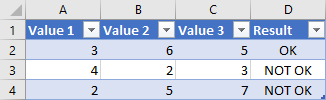﻿ IF multiple conditions - combined with AND and OR in Excel

# IF multiple conditions - combined with AND and OR in Excel

Hello, I would like to create an excel formula with IF, AND and OR conditions. Until now I didn't use OR, so I used the nested condition and it looked like =IF(A2>5,IF(B2>5,"OK","NOT OK"),"NOT OK").

Now I am not sure how to include OR condition into the formula above. Do you know how to do it ?
Hi,

To create the formula, you have to combine IF, AND and OR functions. The following formula is more simple and will give the same result as the one you are using:
=IF(AND(A2>5,B2>5),"OK","NOT OK")

In case of more conditions, it is needed only to add values into AND function. For example:
=IF(AND(A2>5,B2>5,C2>5,D2>5),"OK","NOT OK")

To add OR condition, you need to include OR function inside AND function. The formula could look like:
=IF(AND(A2>2,OR(B2>5,C2>5)),"OK","NOT OK")

The results will be:You can also include AND function inside OR function when needed.

Related questions